# 开关变换器优缺点

## 优点

1. 能够达到最高的效率
2. 可以实现多种不同的拓扑结构：

$V_O>V_{IN}\Rightarrow(Boost)$

$V_O

$V_{IN_min}

$负压输出\Rightarrow(反极性)$

1. 可实现隔离变换
2. 可满足更多设计的需求

1. 在系统中引入了磁场
2. 不可避免的开关噪声
3. 是比较复杂的解决方案

# 板上电源常见的非隔离拓扑

## 输出总是低于输入

$V_{OUT}=V_{IN} \times D$

## 输出总是高于输入

$V_{OUT}=V_{IN} \div (1-D)$

## 输出可能低于输入，也可能高于输入

$V_{OUT}=-V_{IN} \times \frac{D}{1-D}$

$V_{OUT}=V_{IN} \times D \\ \textbf{or} \\ V_{OUT}=V_{IN} \div (1-D)$

# Buck电路

## Buck电路构成

• Buck变换器又称降压电路，其基本拓扑如图所示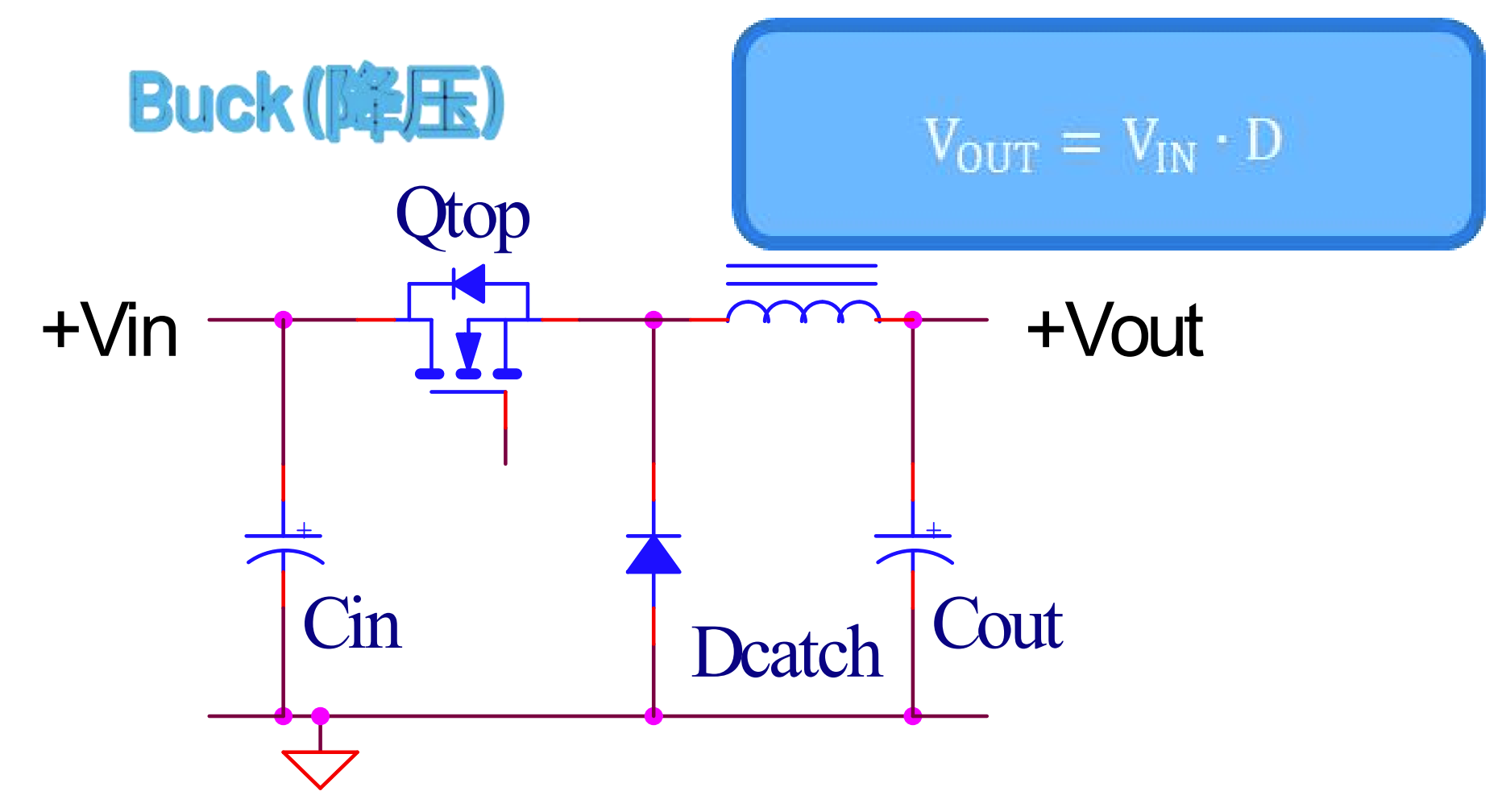## Buck工作原理

• Buck变换器工作在电感电流连续模式下的工作原理如下

• 开关管的导通与关断受控制电路输出的驱动脉冲控制。当控制电路脉冲输出高电平时，开关管导通续流二极管D阳极电压为零，阴极电压为电压电压Us，因此反向截止，开关上流过电流is流经电感L向负载R供电；此时L中的电流逐渐上升，在L两端产生左端正右端负的自感电势阻碍电流上升，L将电能转化为磁能存储起来。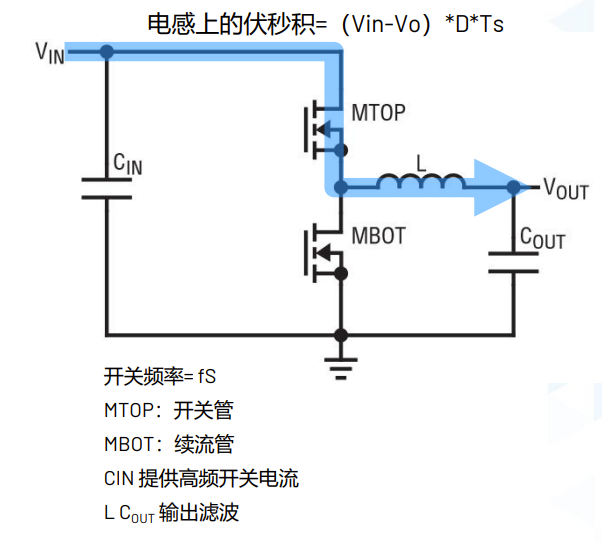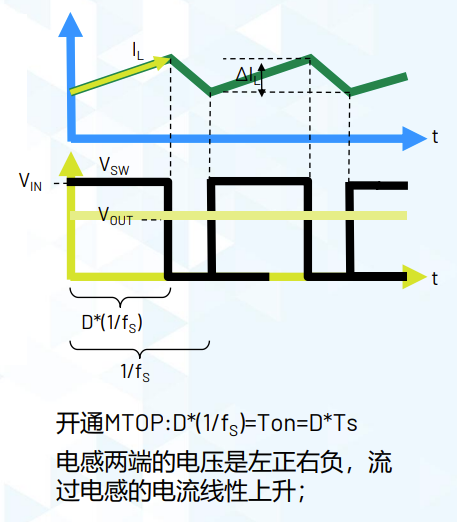• 经过时间ton后，控制电路脉冲为低电平，开关管关断，但L中的电流不能突变，这时电感L两端产生右端正左端负的自感电势阻碍电流下降，从而使D正向偏置导通，于是L中的电流经D构成回路，电流值逐渐下降，L中储存的磁能转化为电能释放出来供给负载R。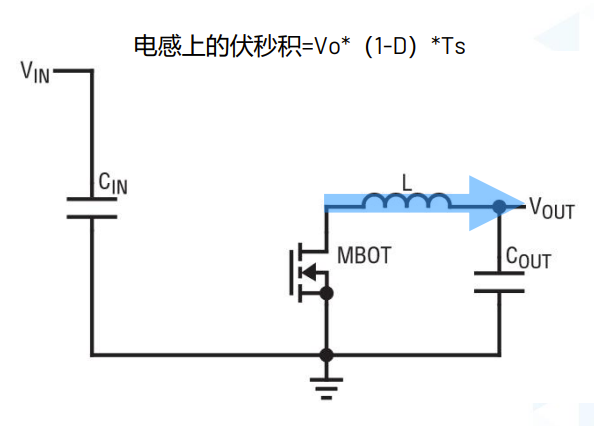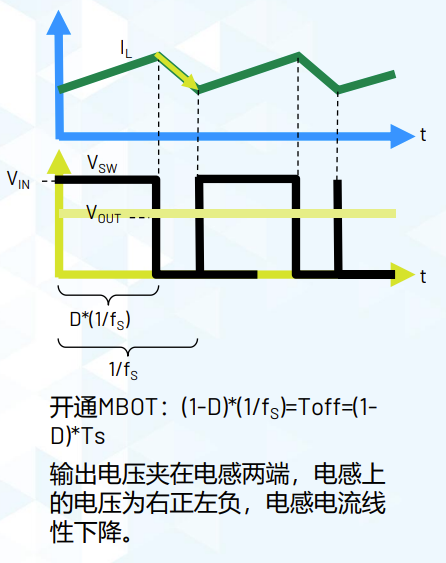• 经过时间tof后，控制电路脉冲又使开关管导通，重复上述过程，即可实现电路的降压。

• 在稳态、连续（CCM）、器件理想的情况下，电感电流在开通和关断电流变换相等，开通关断伏秒积平衡，$V_O=V_{IN}*D$

• 需要注意：

1. 滤波电容C的作用是为了降低输出电压Uo的脉动。
2. 续流二极管D是必不可少的元件，若无此二极管，电路不仅不能正常工作，而且在开关管由导通变为关断时，L两端将产生很高的自感电势从而损坏开关管。

## Buck数学公式

• 开通关断伏秒积平衡：

$V_{OUT} = V_{IN} \cdot D ; D=t_{ON} \cdot f_S$

• 电感上的纹波电流：

$\Delta I_{L} = \frac{V_{OUT} \cdot (1-V_{OUT}/V_{IN})}{L\cdot f_S}$

• 可推得所需电感值：

$L =\frac{V_{OUT} \cdot (1-V_{OUT}/V_{IN})}{\Delta I_{L}\cdot f_{S}}$

• 电感上的最大电流：

$I_{L(MAX)} = I_{OUT} + 0.5\cdot \Delta I_{L}$

• 输出电压纹波：

$V_{RIPPLE}= \Delta I_{L} \cdot (ESR_{C_{OUT}}+ \frac{1}{8f_sC_{OUT}})$

• 输入电容纹波电流：

$I_{C_{IN}(RMS)}=\frac{I_{OUT(MAX)}}{\eta}(\sqrt{D(1-D)})$

## 非同步vs.同步Buck

### 非同步Buck

• 非同步Buck使用肖特基二级管作为续流管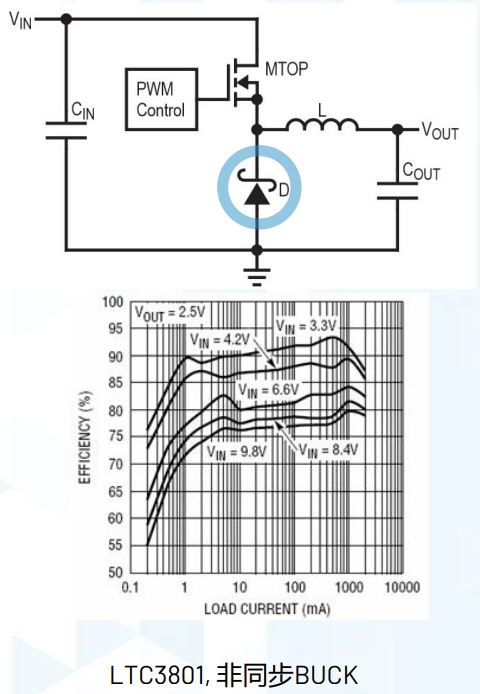### 同步Buck

• 同步Buck电路采用通态电阻极低的专用功率MOSFET，来取代整流二极管作为续流管
• 同步Buck能够大大提高DC/DC的变换效率且不存在由于肖特基二极管势垒电压而造成的死区电压。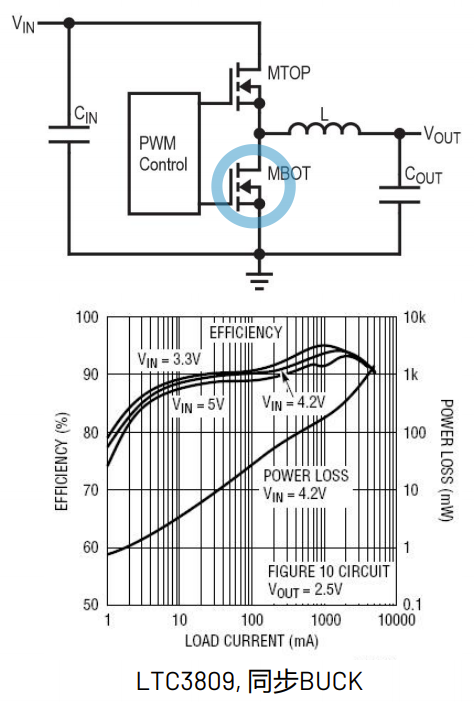# Boost电路

## Boost电路构成

• Boost变换器又称升压电路，其基本拓扑如图所示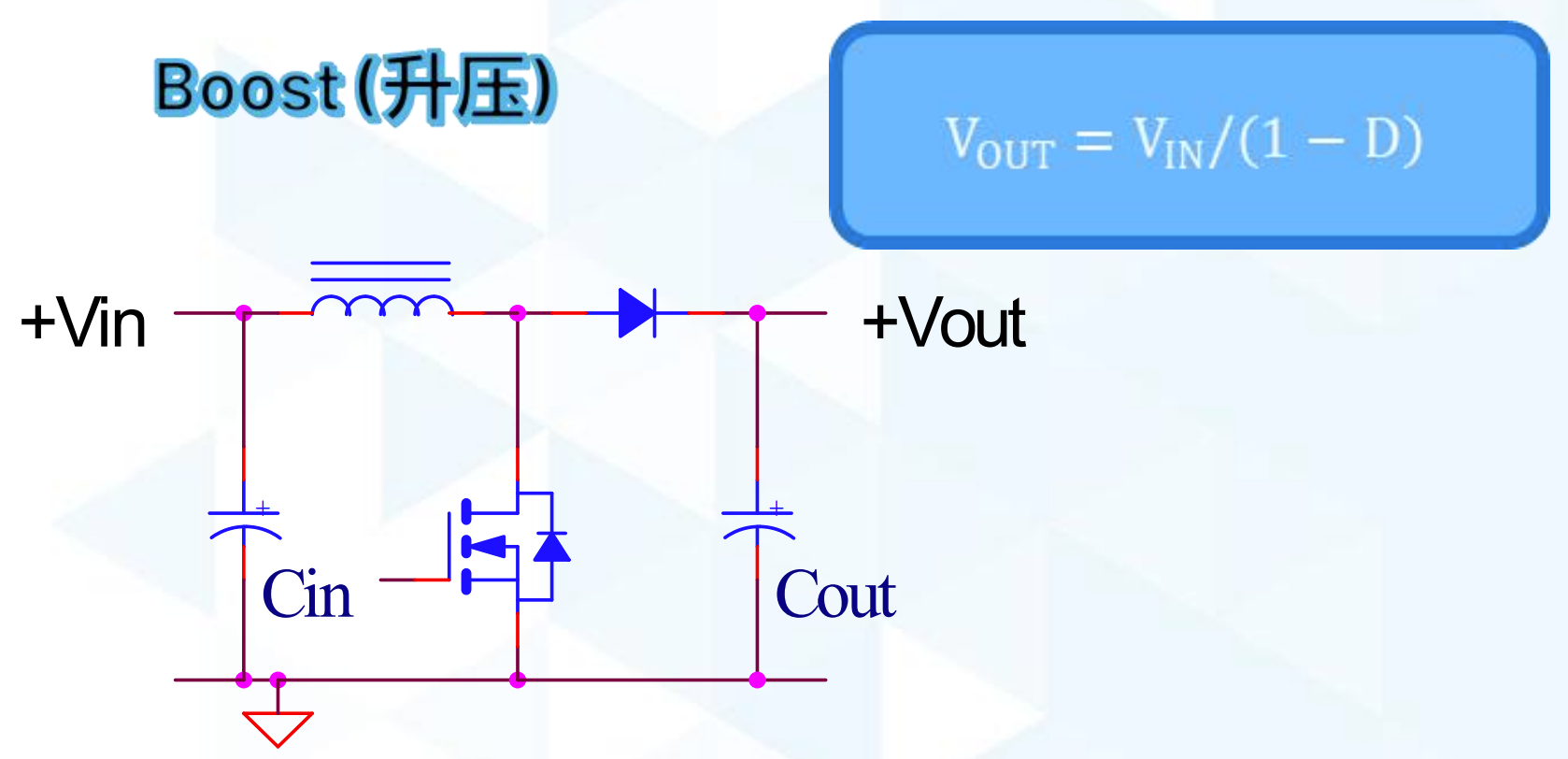## Boost工作原理

• Boost变换器工作在电感电流连续模式下的工作原理如下
• 在充电过程中，开关闭合（三极管导通），等效电路图入下图所示，开关（三极管）处用导线代替。这时，输入电压流过电感。二极管防止电容对地放电。由于输入是直流电，所以电感上的电流以一定的比率线性增加，这个比率跟电感大小有关。随着电感电流增加，电感里储存了一些能量。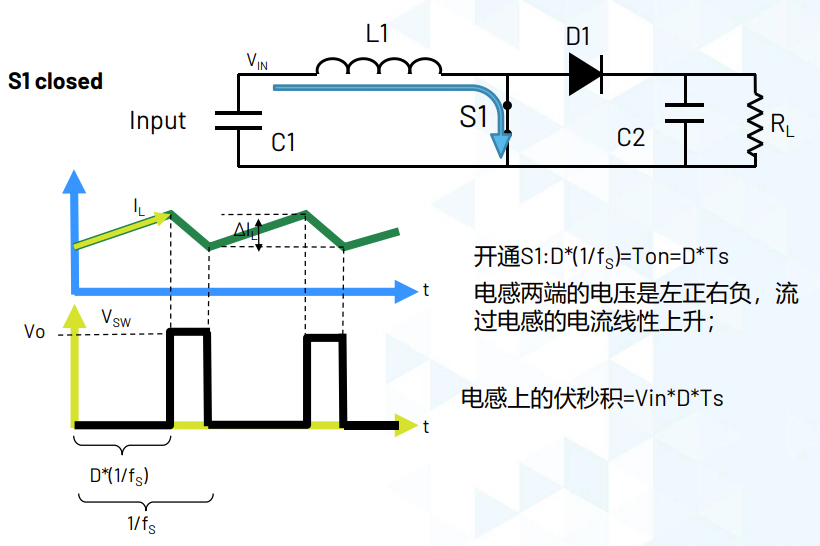• 当开关断开（三极管截止）时，由于电感的电流保持特性，流经电感的电流不会马上变为0，而是缓慢的由充电完毕时的值变为0。而原来的电路已断开，于是电感只能通过新电路放电，即电感开始给电容充电，电容两端电压升高，此时电压已经高于输入电压了。升压完毕。升压过程就是一个电感的能量传递过程。充电时，电感吸收能量，放电时电感放出能量。如果电容量足够大，那么在输出端就可以在放电过程中保持一个持续的电流。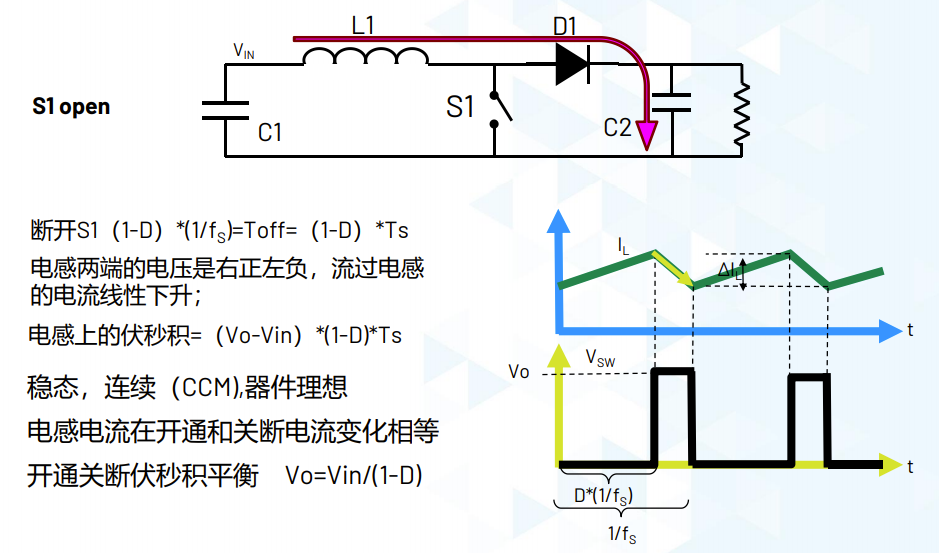• 这个通断的过程不断重复，就可以在电容两端得到高于输入电压的电压。
• 在稳态、连续（CCM）、器件理想的情况下，电感电流在开通和关断电流变换相等，开通关断伏秒积平衡，$V_O=V_{IN}/(1-D)$
• 需要注意：
1. 滤波电容C的作用是为了降低输出电压Uo的脉动。
2. 续流二极管D是必不可少的元件，若无此二极管，电路不仅不能正常工作，而且在开关管由关断变为导通时，电容C两端的电压会通过MOS管对地短路，从而放出积累的电荷。

## Boost数学公式

• 开通关断伏秒积平衡：

$V_{OUT} = \frac{V_{IN}}{1-D} ; D=\frac{1-V_{IN}}{V_{OUT}}$

• 电感上的纹波电流：

$\Delta I_{L} =\frac{V_{IN}}{L\cdot T_{ON}}= \frac{V_{IN} \cdot (1-V_{IN}/V_{OUT})}{L\cdot f_S}$

• 可推得所需电感值：

$\Delta I_{L} = \frac{V_{IN} \cdot (1-V_{IN}/V_{OUT})}{\Delta I_L\cdot f_S}$

• 电感上的最大电流：

$I_{L(MAX)} = I_{OUT} + 0.5\cdot \Delta I_{L}$

• 输出电容纹波电流：

$I_{C_{OUT}(RMS)}=I_{OUT(MAX)}\cdot(\sqrt{D/(1-D)})$

## Boost电路特点

• 因为二极管D1的存在，即使是同步整流，也有体二极管存在，那么带来的问题：
1. 输出不能短路；
2. 输入，输出不能彻底断开，存在输入到输出的漏电路径。即，即使在关闭Boost电路时，电路仍会输出与输入电压相同的一个电压值。

# 升降压同步BUCK-BOOST

• 对于实际的使用场景，我们可能需要一个技能格升压又可以降压的升降压电路。如锂电池2.8V-4.3V， 输出3.3V。

## BUCK-BOOST工作原理

• 升降压同步BUCK-BOOST电路，结合了同步BUCK和同步BOOST的工作原理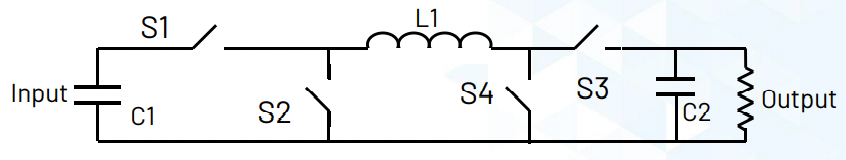1. 降压模式工作(Vin>Vo)：S3始终闭合， S4始终断开；S1/S2互补导通，和BUCK工作模式完全一样。
2. 升压模式工作(Vin<Vo)：S1始终闭合，S2始终断开。S4/S3互补导通，和BOOST工作模式完全一样。
3. 升降压工作模式 (Vin接近Vout) ：S1/S2/S3/S4全部工作在开关状态。